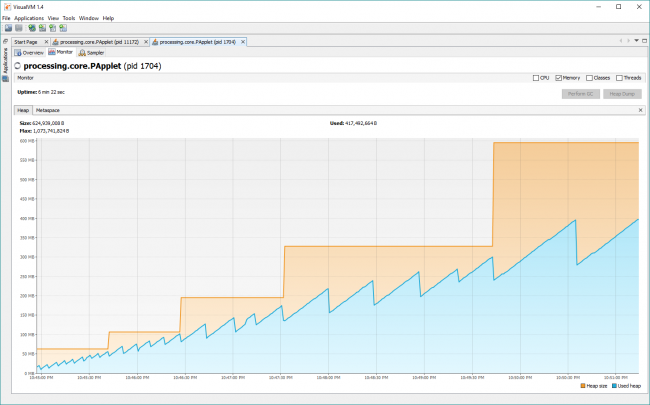#### Howdy, Stranger!

We are about to switch to a new forum software. Until then we have removed the registration on this forum.

# PShape rotations and translations increase memory used (used heap and heap size) with time

edited March 2018

I created some cool planetary gears with vectors and shapes (not PShapes) with success!

Demo here: https://youtu.be/RTZvgVu4LeU

I tried replacing the gear shapes with PShapes and when I do rotations or translations the memory used (used heap and heap size) keeps growing!

I used Visual VM to monitor and included a screen grab.

Why does memory grow when I rotate or translate PShapes? Is this a memory leak? Seems fine if I comment out the translations and rotations.

Sample code is something I was testing and a demo of growing heap:

``````// Author:      GLV
// Date:        2018-03-04
// Version:     04

PShape s2;
int time_start;
PVector v1;

void settings()
{
size(600, 600, P2D);
}

void setup()
{
s2 = createShape();
s2.beginShape();
fill(102);
stroke(255);
strokeWeight(0);
s2.vertex(0, 0);
s2.vertex(0, 20);
s2.vertex(20, 20);
s2.vertex(20, 0);
s2.endShape(CLOSE);
time_start = millis();
v1 = new PVector(0, 0);
}

void draw()
{
background(0);
translate(width/2, height/2);
noFill();
strokeWeight(3);
v1.set(0, 20*2*40/TAU);
for (int i=0; i<=40; i++)
{
fill(255, 255, 0);
v1.rotate(TAU/40);
s2.translate(-10, -10);    //Comment this line
shape(s2, v1.x, v1.y);
s2.translate(10, 10);         //Comment this line
}

if((millis()-time_start) >= 10000)
{
//      System.gc();
//      Runtime.getRuntime().gc();
time_start = millis();
println("Garbage Collection");
}
}
``````Tagged:

• You could try commenting out lines to try and pinpoint the culprit

• As I stated: "Seems fine if I comment out the translations and rotations."

I added "// Comment this line" to code.

I have had similar issues with PShapes with other code but only when I rotate or translate.

Are there any know issues with PShapes that I need to be aware of?

• edited March 2018 Answer ✓

I have solved my PShape memory growing issues in the original example I provided:

``````    s2.translate(-10, -10);
shape(s2, v1.x, v1.y);
//    s2.rotate(-v1.heading());  //Commented out; replaced with resetMatrix()
//    s2.translate(10, 10);      //Commented out; replaced with resetMatrix()
s2.resetMatrix();
``````

Learning something new every day! :)

• @GLV Just curious if pushMatrix/popMatrix could do the trick for you as well instead of resetting. I don't think resetting to be a good absolute solution as you might need to conserve some of those transformations... That is what I foresee in certain situations.

Kf

• Your planetary gear vizualisation is really very nice.

• Thanks everyone for comments and feedback! @koogs @kfrajer @Moxl

Some test code (below) I wrote to explore a few different ways of building my gears.

The most complicated was my first attempt building them with vectors! I learned a lot along the way...

``````// Author:      GLV
// Date:        2018-03-07
// Description: Gear Maker using vectors, shapes and PShapes.
// This is just "test" code!
// Version:     2

PShape s2;
PVector v1, v2, v3, v4, v5;
int time_start;
int count = 5;
int numgears = 5;
float th = 0;
int i;

void settings()
{
size(1280, 1024, P3D);
}

void setup()
{
s2 = createShape();
s2.beginShape();
s2.strokeWeight(3);   //strokeWeight() can only be called between beginShape() and endShape()
s2.vertex(0, 0);
s2.vertex(0, 20);
s2.vertex(20, 20);
s2.vertex(20, 0);
s2.endShape(CLOSE);

v1 = new PVector(0, 0);
v2 = new PVector(0, 0);
v3 = new PVector(0, 20);
v4 = new PVector(20, 20);
v5 = new PVector(20, 0);
time_start = millis();
}

void draw()
{
background(0);

numgears = count;

v1.set(1,0);

for (i=0; i<numgears; i++)
{
switch (1)    //switch for future use
{
case (1):
shapes01();
pshapes01();
pshapes02();
pshapes03();
}

v1.rotate(TAU/numgears);

// angle from incrementing
th += TAU/numgears;

// heading:  0 to 180, -180 to 0
// theta:    0 to 180, 180 to 360
println("theta:   " + round(th/TAU*360));
println(" ");
}

th = 0;

if((millis()-time_start) >= 1000)
{
time_start = millis();
count++;
if (count >=40) count = 5;
}
}

// Build gear tooth with vectors and place tooth at end of vector
void shapes01()
{
float xt = 10;
float yt = 10;
v2.set(0-xt, 0-yt);
v3.set(0-xt, 20-yt);
v4.set(20-xt, 20-yt);
v5.set(20-xt, 0-yt);

pushMatrix();
translate(1*width/4, height/4);
lines();                          //draw vector line and ellipse

fill(255, 0, 0);
beginShape();
vertex(v2.x, v2.y); vertex(v3.x, v3.y);
vertex(v3.x, v3.y); vertex(v4.x, v4.y);
vertex(v4.x, v4.y); vertex(v5.x, v5.y);
vertex(v5.x, v5.y); vertex(v2.x, v2.y);
endShape(CLOSE);
popMatrix();
}

// Build gear tooth with PShape and place tooth at end of vector
//  s2.translate() and s2.rotate() PShape s2
void pshapes01()
{
pushMatrix();
translate(3*width/4, height/4);
lines();                          //draw vector line and ellipse

s2.setFill(color(0, 255, 0));
s2.setStroke(color(128));
s2.translate(-10, -10);
shape(s2, v1.x, v1.y);
s2.resetMatrix();
popMatrix();
}

// Build gear tooth with PShape and place tooth at end of vector
// translate() and rotate() PShape s2
void pshapes02()
{
pushMatrix();
translate(1*width/4, 3*height/4);
lines();                          //draw vector line and ellipse

s2.setFill(color(0, 255, 255));
s2.setStroke(color(128));
translate(v1.x, v1.y);
shape(s2, 0-10, 0-10);
popMatrix();
}

// Build gear tooth with box() and place at end of vector
// translate() and rotate() box
void pshapes03()
{
pushMatrix();
translate(3*width/4, 3*height/4);
lines();                          //draw vector line and ellipse

fill(color(255, 255, 0));
stroke(128);
translate(v1.x, v1.y);
rotate(v1.heading());    //can use this or below!
//  rotate(th);
box(20, 20, 0);
popMatrix();
}

// Draw vector line and ellipse
void lines()
{
stroke(128);
strokeWeight(2);
line(0, 0, v1.x, v1.y);
noFill();
}
``````
• ``````//if ((millis()-time_start) >= 1000)
//{
//  time_start = millis();
//  count++;
//  if (count >=40) count = 5;
//}

count=int(map(mouseX,0,width,3,40));
``````

Kf

• @kfrajer I liked that!